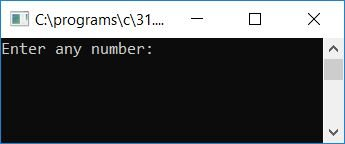# C Program to Find the Total Number of Digits in a Given Number

In this article, we will learn to create a program in C that will ask the user to enter any number (at run-time) to find and print the total number of digits present in that given number. Here is the program:

```#include<stdio.h>
#include<conio.h>
int main()
{
int num, tim=0;
printf("Enter any number: ");
scanf("%d", &num);
while(num>0)
{
tim++;
num = num/10;
}
printf("\nTotal number of Digit = %d", tim);
getch();
return 0;
}```

As the above program was written in the Code::Blocks IDE, here is the output you will also get on your output screen after a successful build and run. This is the first snapshot of the sample run:Supply any number, say 24304, and press ENTER to see the output. As the number 24304 has a total of 5 digits, here is the output you will get:Here are some of the main steps used in the above program:

• Receive any number as input.
• Create a while loop that runs until the number holds a value that is greater than 0.
• Inside the while loop, increment a variable, say tim, that is responsible for counting the total number of digits present inside the given number.
• Never forget to initialize the variable tim with 0 at the start of the program inside the main() function.
• After incrementing the value of the variable tim inside the while loop, divide the given number by 10 for the next check and increment.
• In this way, after exiting from the while loop, the variable tim holds the value that will be the value of the total number of digits present in the given number.

Before closing the discussion on this post, I'm willing to include one more example that does the same job of finding the total number of digits in a given number. I used comments to describe the main codes used in the following C program:

C Code
```#include <stdio.h>

int main() {
int num, count = 0;         // declare variables for the number and count of digits

printf("Enter a number: "); // ask the user to input a number
scanf("%d", &num);          // read the input number from the user

while (num != 0) {          // loop until the number is completely divided
count++;                // increment the count of digits
num /= 10;              // divide the number by 10 to remove the last digit
}

printf("Total number of digits: %d\n", count);   // print the total number of digits

return 0;
}```
Output
```Enter a number: 987654321
Total number of digits: 9```

C Quiz

« Previous Program Next Program »